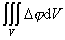[高斯公式]RdV=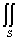RdS=RNdS式中为空间区域的边界曲面，NS上一点的法线单位矢量，R(r)=(X(x, y,z),Y(x, y,z),Z(x, y,z))

VS上有连续偏导数.

[斯托克斯公式]rot dSrot RNdSRdr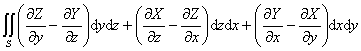==[格林公式]dS=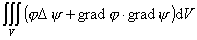dS=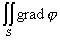dS=